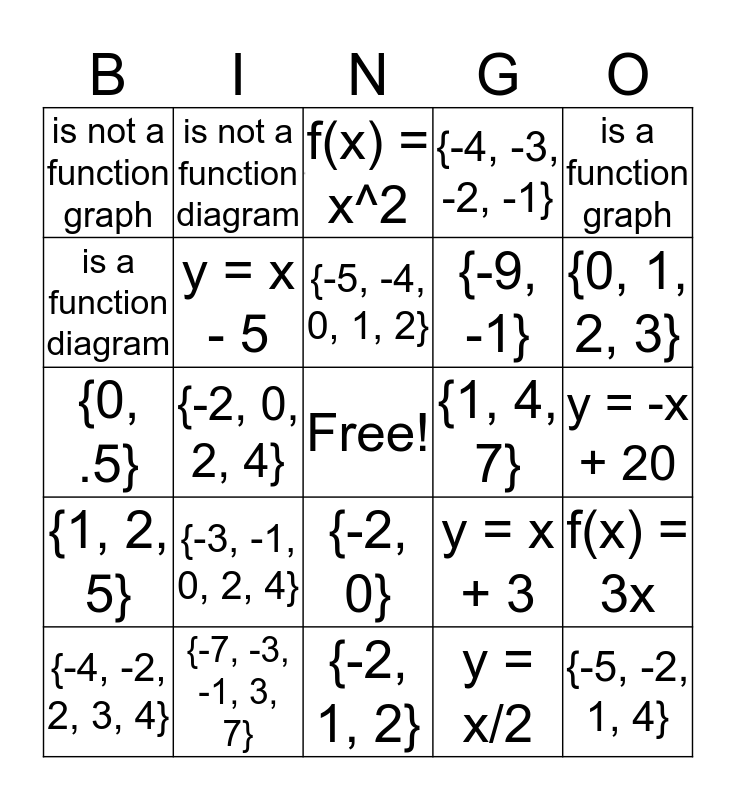# Alg 1A 5-1 to 5-4 Practice A ReviewThis bingo card has 1 images, a free space and 24 words: {-3, -1, 0, 2, 4}, {-7, -3, -1, 3, 7}, {-5, -4, 0, 1, 2}, {-4, -2, 2, 3, 4}, is a function graph, is not a function graph, is a function diagram, is not a function diagram, {1, 4, 7}, {-2, 0}, {-9, -1}, {0, .5}, y = x + 3, f(x) = 3x, y = x - 5, y = x/2, y = -x + 20, f(x) = x^2, {0, 1, 2, 3}, {-2, 0, 2, 4}, {-5, -2, 1, 4}, {1, 2, 5}, {-4, -3, -2, -1} and {-2, 1, 2}.

## Play Online